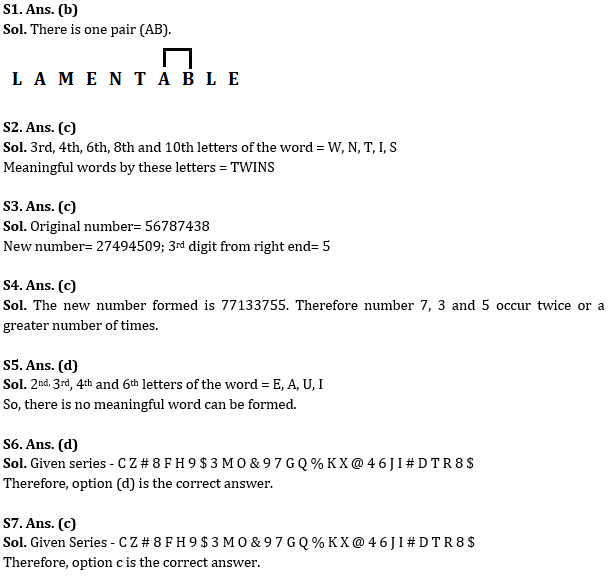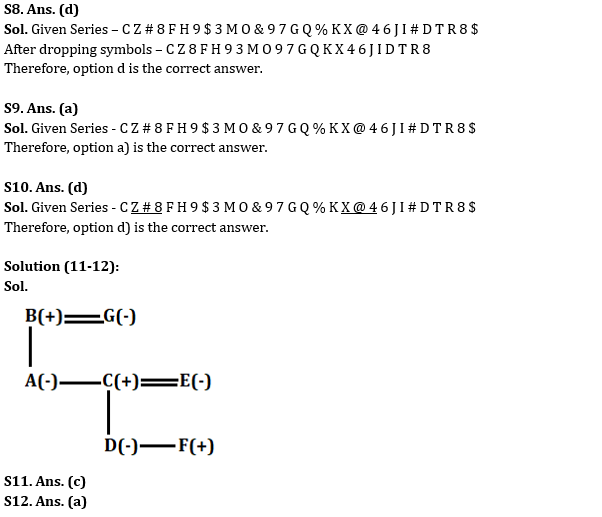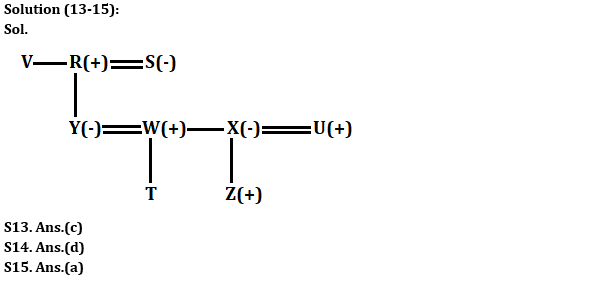Latest Banking jobs   »

# Reasoning Ability Quiz For SBI Clerk Prelims 2022- 31st May

Q1. How many such pairs of letters are there in the word ‘LAMENTABLE’ each of which has as many letters between them in the word as in the English alphabet (Both forward and backward direction)?
(a) None
(b) One
(c) Two
(d) Three
(e) None of these

Reasoning Ability Quiz For SBI Clerk Prelims 2022 31st May check in hindi

Q2. If it is possible to make a meaningful word with the third, fourth, sixth, eighth and tenth letters of the word DOWNSTAIRS, then which of the following will be the first letter of that meaningful word? If no meaningful word can be made, give ‘X’ as the answer. If more than one meaningful word can be made, give ‘M’ as the answer.
(a) W
(b) S
(c) T
(d) X
(e) M

Q3. If in the number 56787438, 1 is added to each even digit and 3 is subtracted from each odd digit, then which digit will be 3rd from the right end?
(a) 4
(b) 9
(c) 5
(d) 0
(e) 7

Q4. If ‘2’ is subtracted from each odd digit and ‘1’ is added to each even digit in the number 96352947, then how many numbers occur twice or more in the number thus formed?
(a) One
(b) Two
(c) Three
(d) Four
(e) None

Q5. If it is possible to make a meaningful word with the second, third, fourth, and sixth letters of the word BEAUTIFUL, then which of the following will be the second letter of that meaningful word? If no meaningful word can be made, give ‘X’ as the answer. If more than one meaningful word can be made, give ‘Y’ as the answer.
(a) I
(b) E
(c) A
(d) X
(e) Y

Directions (6-10): Study the following alphanumeric- symbol series carefully and answer the questions accordingly.

C Z # 8 F H 9 \$ 3 M O & 9 7 G Q % K X @ 4 6 J I # D T R 8 \$

Q6. What should come in place of question mark in the following series?
ZF#    9M\$    &G9    %@K   ?
(a) JDI
(b) 4I6
(c) 6IJ
(d) 6#J
(e) None of these

Q7. How many such numbers are there in the given series which are immediately preceded or followed by a consonant?
(a) Three
(b) Four
(c) Mora than five
(d) Five
(e) None of these

Q8. If all the symbols are dropped from the series, then which element will be fifth to the right of the one which is fourteenth from the right end of the new arrangement?
(a) G
(b) K
(c) 4
(d) X
(e) None of these

Q9. Which of the following element is 17th to the right of the one which is 24th from the right end of the given arrangement?
(a) I
(b) J
(c) #
(d) T
(e) None of these

Q10. How many such symbols are there in the given series which are immediately preceded by consonant but not immediately followed by consonant?
(a) Four
(b) One
(c) Three
(d) Two
(e) None of these

Directions (11-12): Study the information given below and answer the questions based on it.
Seven people A, B, C, D, E, F and G belong to the same family. B is the father of A. C is the father of D. E is the mother of F. D is the sister of F. G is the mother of C. A is the sister of C. E has only one daughter.

Q11. How is F related to G?
(a) Son
(b) Daughter
(c) Grandson
(d) Grand-daughter
(e) Brother

Q12. How is A related to E?
(a) Sister-in-law
(b) Sister
(c) Mother
(d) Aunt
(e) Daughter
(e) None of these

Directions (13-15): Study the information carefully and answer the questions accordingly.
There are nine members in a family of three generations. R is the grandfather of T and married to S. U is the brother-in- law of W. Y is the niece of V who is the sibling of R. Z is the nephew of W. W and X are sibling and both are married. R has only one daughter. T and Z are cousins. V is unmarried person.

Q13. How W is related to T?
(a) Nephew
(b) Son
(c) Father
(d) Uncle
(e) None of these

Q14. Find the odd one.
(a) X, Z
(b) W, S
(c) R, U
(d) V, Y
(e) Y, X

Q15. Who among the following is a married couple?
(a) X, U
(b) Y, X
(c) U, W
(d) V, T
(e) None of these

Solutions#### Congratulations!